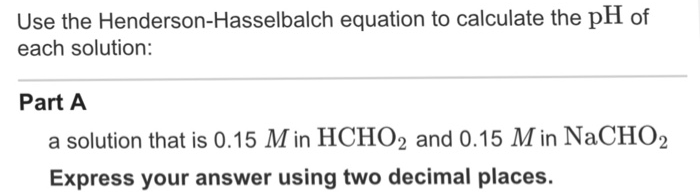# Problem: Use the Henderson-Hasselbalch equation to calculate the pH of each solution: A solution that is 0.15 Min HCHO2 and 0.15 Min NaCHO2 Express your answer using two decimal places.

###### FREE Expert Solution

Using the Henderson-Hasselbalch equation, we can find the pH of the acid (HCHO2) conjugate base (NaCHO2)

96% (248 ratings)###### Problem Details

Use the Henderson-Hasselbalch equation to calculate the pH of each solution: A solution that is 0.15 Min HCHO2 and 0.15 Min NaCHO2 Express your answer using two decimal places.